C. Gourmet Cat（Mod思维题）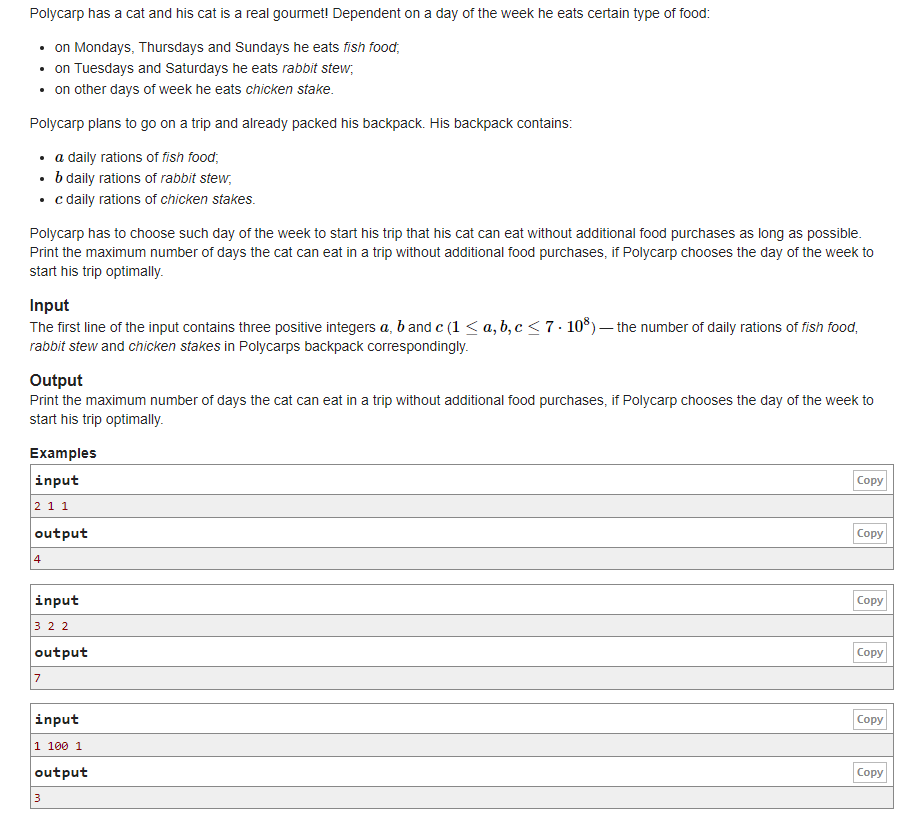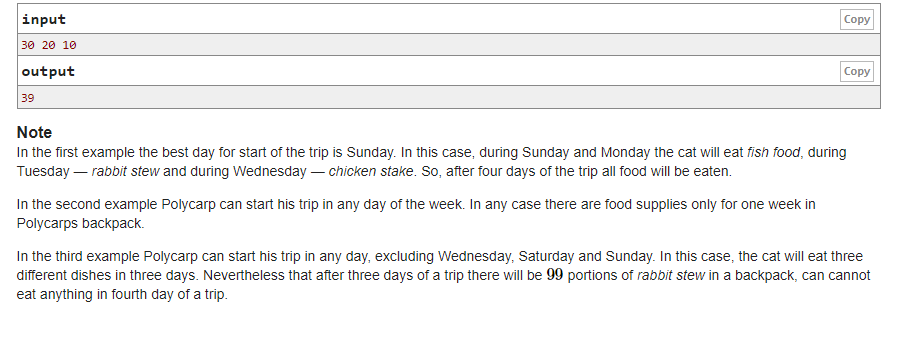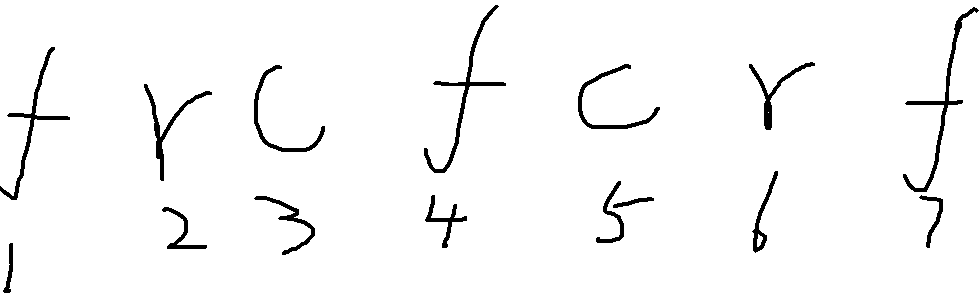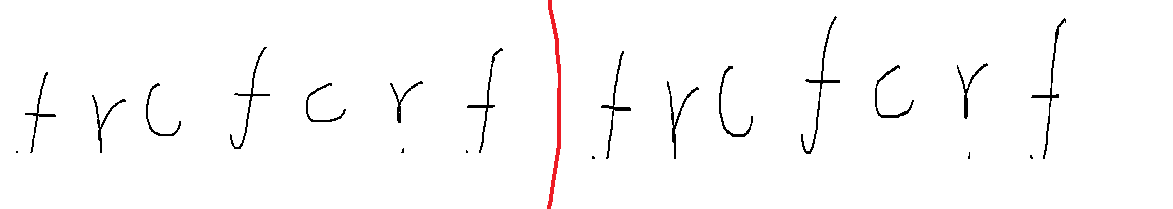QAQ;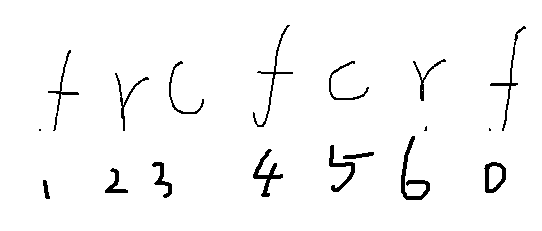AC代码：

#include <bits/stdc++.h>
using namespace std;
typedef long long ll;
int main(){

ll a,b,c;
scanf("%lld %lld %lld",&a,&b,&c);
ll Min=min(a/3,min(b/2,c/2));
ll ans=Min*(3+2+2);
a-=Min*3;
b-=Min*2;
c-=Min*2;
ll f=a,r=b,cc=c;
ll Max=0,t=0;
for(int i=1;i<=7;i++){

t=0;
f=a;r=b;cc=c;
for(int j=0;j<=6;j++){

int tt=(i+j)%7;
if(tt==0||tt==1||tt==4){

if(f){

f--;t++;
}else break;
}
if(tt==2||tt==6){

if(r){

r--;t++;
}else break;
}
if(tt==3||tt==5){

if(cc){

cc--;t++;
}else break;
}
}
Max=max(t,Max);
}
printf("%lld\n",ans+Max);
return 0;
}